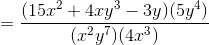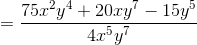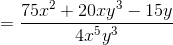## Example Questions

### Example Question #8 : Rational Expressions

Simplify (4x)/(x– 4) * (x + 2)/(x– 2x)

x/(x – 2)2

(4x+ 8x)/(x+ 8x)

4/(x + 2)2

x/(x + 2)

4/(x – 2)2

4/(x – 2)2

Explanation:

Factor first.  The numerators will not factor, but the first denominator factors to (x – 2)(x + 2) and the second denomintaor factors to x(x – 2).  Multiplying fractions does not require common denominators, so now look for common factors to divide out.  There is a factor of x and a factor of (x + 2) that both divide out, leaving 4 in the numerator and two factors of (x – 2) in the denominator.

### Example Question #9 : Rational Expressions

what is 6/8 X 20/3

18/160
5
9/40
120/11
3/20
Explanation:

6/8 X 20/3 first step is to reduce 6/8 -> 3/4 (Divide top and bottom by 2)

3/4 X 20/3 (cross-cancel the threes and the 20 reduces to 5 and the 4 reduces to 1)

1/1 X 5/1 = 5

### Example Question #10 : Rational Expressions

Evaluate and simplify the following product: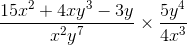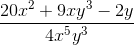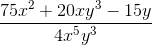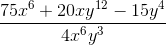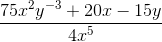Explanation:

The procedure for multplying together two rational expressions is the same as multiplying together any two fractions: find the product of the numerators and the product of the denominators separately, and then simplify the resulting quotient as far as possible, as shown: